Like   Tweet   +1   in## Friday, July 17 --- 7:15 - 9:30PM

 table div table+table+table div table{width:100%;padding:0}table div table+table+table div table img{width:96.23%;padding:0;float:none}table div table+table+table div table td{width:100%;padding:0 1.88% 18px}/* styles *//* styles */ Welcome to Friday night LIVE at All Saints! We are back in the Abbot Rd parking lot on the West side of All Saints church. This month we have Zach Henrys, Reuben Stump and Taylor Herrmann, Jim Jersey, Bill Walbridge, Kerby Rials, Janet Colson, Austin Kaufmann, Johnny Zawacki and Andrew Pommerville. PLEASE NOTE: BRING YOUR OWN CHAIR, FOOD AND DRINK...THE CHURCH RESTROOMS WILL BE UNAVAILABLE....Bagpipes start us off at 7:15pm!!
 table div table+table+table+table+table div table{width:100%;padding:0}table div table+table+table+table+table div table img{width:96.23%;padding:0;float:none}table div table+table+table+table+table div table td{width:100%;padding:0 1.88% 18px}/* styles */## Reuben Stump and Taylor Herrmann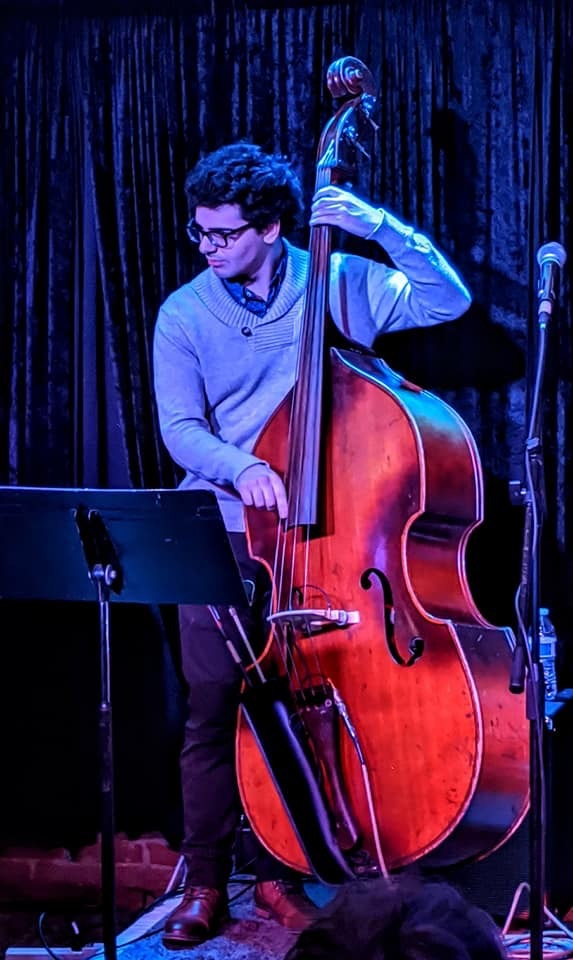Reuben Stump

Taylor Herrmann and Reuben Stump are both musicians from Lansing, and have been performing together in Southeast Michigan for a little under a year. They love to play Jazz Standards and songs from the great American songbook. Taylor is at Oakland University studying Vocal Performance and Anthropology, and Reuben is at U of M in the Jazz Studies program. Reuben might bust out a uke tune too.

 table div table+table+table+table+table+table+table+table div table{width:100%;padding:0}table div table+table+table+table+table+table+table+table div table img{width:96.23%;padding:0;float:none}table div table+table+table+table+table+table+table+table div table td{width:100%;padding:0 1.88% 18px}/* styles */Taylor Herrmann
 table div table+table+table+table+table+table+table+table+table+table div table{width:100%;padding:0}table div table+table+table+table+table+table+table+table+table+table div table img{width:96.23%;padding:0;float:none}table div table+table+table+table+table+table+table+table+table+table div table td{width:100%;padding:0 1.88% 18px}/* styles */## Zach Henrys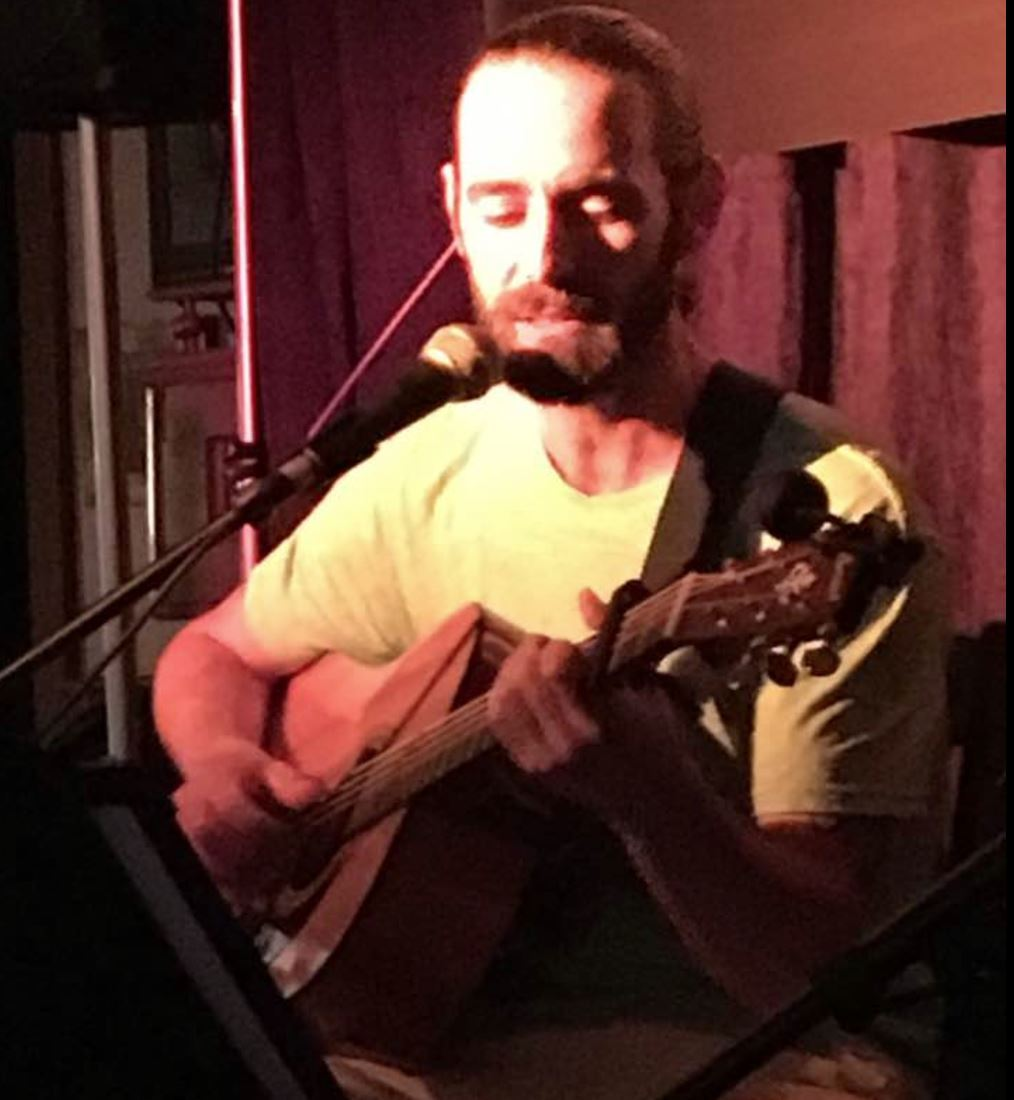Zach Henrys

Zach has been honing his songwriting and guitar-playing skills while being quarantined. He is a thoughtful singer-songwriter who looks forward to the Above The Bridge Songwriter's Weekend (an all inclusive weekend workshop in Curtis, Michigan) but unfortunately it was cancelled like so many things. He feels great to be out performing live again. Welcome back Zach

 table div table+table+table+table+table+table+table+table+table+table+table+table+table div table{width:100%;padding:0}table div table+table+table+table+table+table+table+table+table+table+table+table+table div table img{width:96.23%;padding:0;float:none}table div table+table+table+table+table+table+table+table+table+table+table+table+table div table td{width:100%;padding:0 1.88% 18px}/* styles */## Austin KaufmannAustin Kaufmann

I met Austin at a Colson backyard party where he was rockin' it out with Gerund and The Infinitives. They were great! Now he has his own group, The Dangling Participles (go ahead, ask him) who are also wonderful. Tonight I'm glad he will perform, possibly with a youngun. He is a fine musician, singer, songwriter.

 table div table+table+table+table+table+table+table+table+table+table+table+table+table+table+table+table div table{width:100%;padding:0}table div table+table+table+table+table+table+table+table+table+table+table+table+table+table+table+table div table img{width:96.23%;padding:0;float:none}table div table+table+table+table+table+table+table+table+table+table+table+table+table+table+table+table div table td{width:100%;padding:0 1.88% 18px}/* styles */## Janet Colson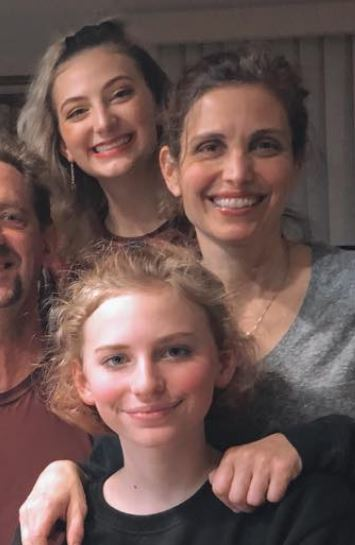Adele, Janet and Fiona Colson

What can you say about this talented family? Performance artists all, they sing, write, dance, act, direct and more. Tonight we'll hear from Janet who might do some comedic reading...or, you never really quite know. Tune in to see/hear her

 table div table+table+table+table+table+table+table+table+table+table+table+table+table+table+table+table+table+table+table div table{width:100%;padding:0}table div table+table+table+table+table+table+table+table+table+table+table+table+table+table+table+table+table+table+table div table img{width:96.23%;padding:0;float:none}table div table+table+table+table+table+table+table+table+table+table+table+table+table+table+table+table+table+table+table div table td{width:100%;padding:0 1.88% 18px}/* styles */## Johnny Zawacki

 table div table+table+table+table+table+table+table+table+table+table+table+table+table+table+table+table+table+table+table+table+table div table,table.module-20{width:50.75%;float:left;padding:0}table div table+table+table+table+table+table+table+table+table+table+table+table+table+table+table+table+table+table+table+table+table div table a{border:0 none;text-decoration:none}table div table+table+table+table+table+table+table+table+table+table+table+table+table+table+table+table+table+table+table+table+table div table img{width:100%!important;border:0 none;text-decoration:none}table div table+table+table+table+table+table+table+table+table+table+table+table+table+table+table+table+table+table+table+table+table div table td{width:100%;padding:0 20px 20px 0}/* styles */ Johnny has been busy during the shutdown...performing online regularly. I've been jealous of all the gear he has to make his performances all the better. He's a regular at Roma's Backdoor and Coffee House On the Square in Owosso as well as Lily Pearls Lounge. He's written some beautiful tunes we may hear tonight.
 table div table+table+table+table+table+table+table+table+table+table+table+table+table+table+table+table+table+table+table+table+table+table div table{width:100%;padding:0}table div table+table+table+table+table+table+table+table+table+table+table+table+table+table+table+table+table+table+table+table+table+table div table img{width:96.23%;padding:0;float:none}table div table+table+table+table+table+table+table+table+table+table+table+table+table+table+table+table+table+table+table+table+table+table div table td{width:100%;padding:0 1.88% 18px}/* styles */## Jim Jersey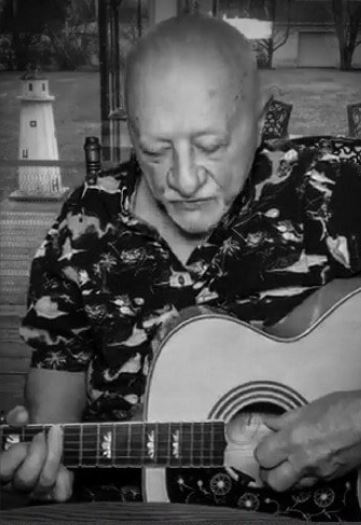Jim Jersey

Our dear Jim Jersey...the greatest Coffeehouse advocate. Jim has been busy performing many times online during the pandemic...bringing his finger-style guitar into your family room. Tonight he'll have a variety of picks from the Great Folk/Pop Tradition (even if they are all in his iPad!)..live and in person. (You can also thank Jim for the shade).

 table div table+table+table+table+table+table+table+table+table+table+table+table+table+table+table+table+table+table+table+table+table+table+table+table+table div table{width:100%;padding:0}table div table+table+table+table+table+table+table+table+table+table+table+table+table+table+table+table+table+table+table+table+table+table+table+table+table div table img{width:96.23%;padding:0;float:none}table div table+table+table+table+table+table+table+table+table+table+table+table+table+table+table+table+table+table+table+table+table+table+table+table+table div table td{width:100%;padding:0 1.88% 18px}/* styles */## Andrew Pomerville

 table div table+table+table+table+table+table+table+table+table+table+table+table+table+table+table+table+table+table+table+table+table+table+table+table+table+table+table div table,table.module-26{width:51.13%;float:left;padding:0}table div table+table+table+table+table+table+table+table+table+table+table+table+table+table+table+table+table+table+table+table+table+table+table+table+table+table+table div table a{border:0 none;text-decoration:none}table div table+table+table+table+table+table+table+table+table+table+table+table+table+table+table+table+table+table+table+table+table+table+table+table+table+table+table div table img{width:100%!important;border:0 none;text-decoration:none}table div table+table+table+table+table+table+table+table+table+table+table+table+table+table+table+table+table+table+table+table+table+table+table+table+table+table+table div table td{width:100%;padding:0 20px 20px 0}/* styles */ Andrew has led quite the interesting life which tonight produced bagpipes! He's the chaplain at Alma College (Home of the Scots!).He's played The Coffeehouse a couple times before (guitar/voice) but it's been a long time. Welcome back Andrew!
 table div table+table+table+table+table+table+table+table+table+table+table+table+table+table+table+table+table+table+table+table+table+table+table+table+table+table+table+table div table{width:100%;padding:0}table div table+table+table+table+table+table+table+table+table+table+table+table+table+table+table+table+table+table+table+table+table+table+table+table+table+table+table+table div table img{width:96.23%;padding:0;float:none}table div table+table+table+table+table+table+table+table+table+table+table+table+table+table+table+table+table+table+table+table+table+table+table+table+table+table+table+table div table td{width:100%;padding:0 1.88% 18px}/* styles */## Kerby Rials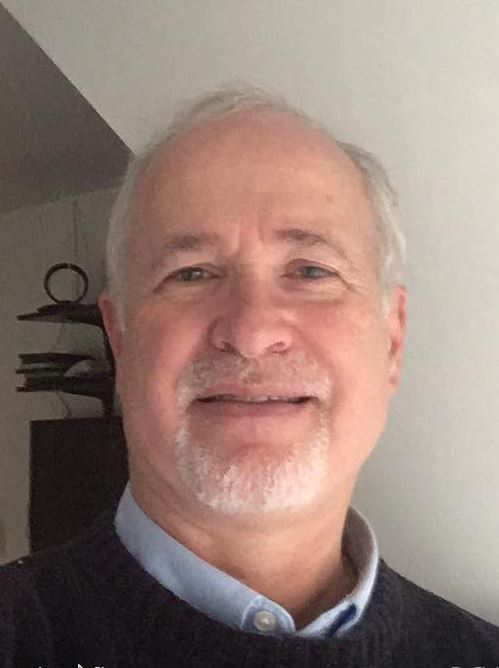Kerby Rials

Kerby is a glad to be back at The Coffeehouse performing live again. He's a fine guitarist who likes to bring the "whole sound" to his performances. There may be guests... Glad you're back, Kerby.

 table div table+table+table+table+table+table+table+table+table+table+table+table+table+table+table+table+table+table+table+table+table+table+table+table+table+table+table+table+table+table+table div table{width:100%;padding:0}table div table+table+table+table+table+table+table+table+table+table+table+table+table+table+table+table+table+table+table+table+table+table+table+table+table+table+table+table+table+table+table div table img{width:96.23%;padding:0;float:none}table div table+table+table+table+table+table+table+table+table+table+table+table+table+table+table+table+table+table+table+table+table+table+table+table+table+table+table+table+table+table+table div table td{width:100%;padding:0 1.88% 18px}/* styles */## Bill Wilbridge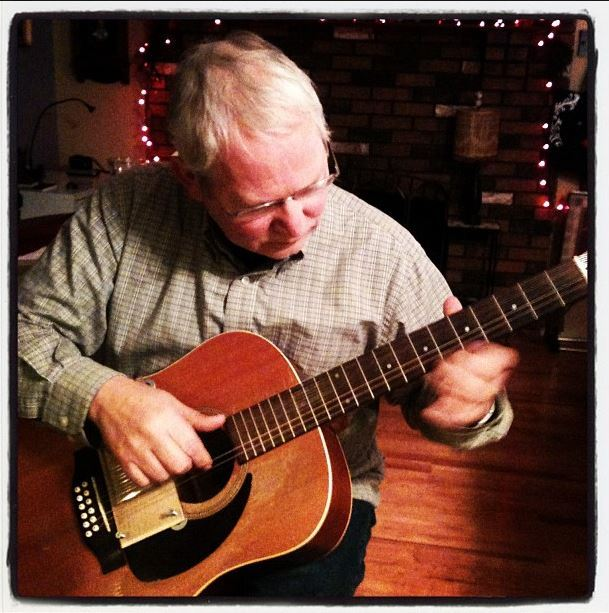Bill Walbridge

A fine musician, Bill is multi-talented. He plays a variety of instruments...all of them well. We have had some wonderful renditions of classic jazz standards as well as classical guitar pieces. Seriously, whayever Bill brings to play, we're going to enjoy it. Nice to have you back Bill

 table div table+table+table+table+table+table+table+table+table+table+table+table+table+table+table+table+table+table+table+table+table+table+table+table+table+table+table+table+table+table+table+table+table+table div table{width:100%;padding:0}table div table+table+table+table+table+table+table+table+table+table+table+table+table+table+table+table+table+table+table+table+table+table+table+table+table+table+table+table+table+table+table+table+table+table div table img{width:96.23%;padding:0;float:none}table div table+table+table+table+table+table+table+table+table+table+table+table+table+table+table+table+table+table+table+table+table+table+table+table+table+table+table+table+table+table+table+table+table+table div table td{width:100%;padding:0 1.88% 18px}/* styles */table div table+table+table+table+table+table+table+table+table+table+table+table+table+table+table+table+table+table+table+table+table+table+table+table+table+table+table+table+table+table+table+table+table+table+table div table{width:100%;padding:0}table div table+table+table+table+table+table+table+table+table+table+table+table+table+table+table+table+table+table+table+table+table+table+table+table+table+table+table+table+table+table+table+table+table+table+table div table img{width:96.23%;padding:0;float:none}table div table+table+table+table+table+table+table+table+table+table+table+table+table+table+table+table+table+table+table+table+table+table+table+table+table+table+table+table+table+table+table+table+table+table+table div table td{width:100%;padding:0 1.88% 18px}/* styles */table div table+table+table+table+table+table+table+table+table+table+table+table+table+table+table+table+table+table+table+table+table+table+table+table+table+table+table+table+table+table+table+table+table+table+table+table div table{width:100%;padding:0}table div table+table+table+table+table+table+table+table+table+table+table+table+table+table+table+table+table+table+table+table+table+table+table+table+table+table+table+table+table+table+table+table+table+table+table+table div table img{width:96.23%;padding:0;float:none}table div table+table+table+table+table+table+table+table+table+table+table+table+table+table+table+table+table+table+table+table+table+table+table+table+table+table+table+table+table+table+table+table+table+table+table+table div table td{width:100%;padding:0 1.88% 18px}/* styles */## 800 Abbot Rd., East Lansing, MI 48823

 /* styles */ Contact Steve Findley, 517-402-2582, steve.findley@gmail.com to perform